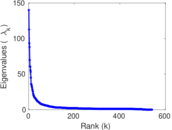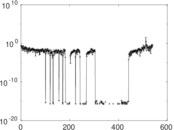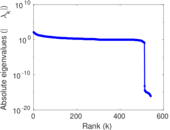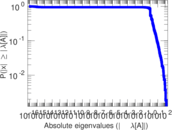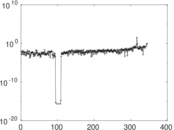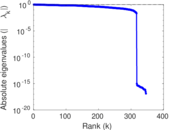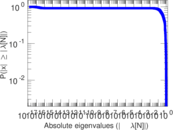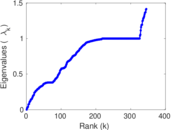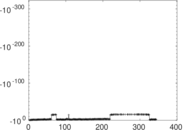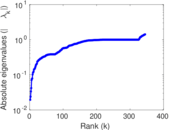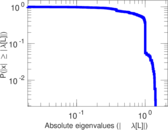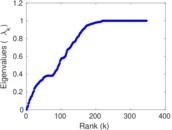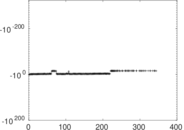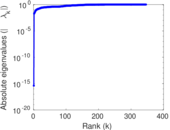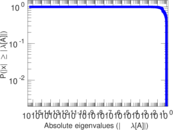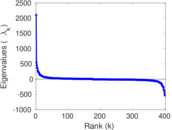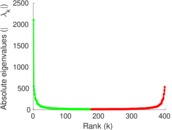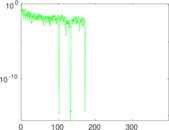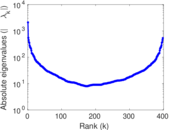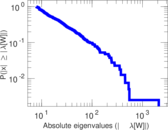# Wikipedia edits (ve)

This is the bipartite edit network of the Venda Wikipedia. It contains users and pages from the Venda Wikipedia, connected by edit events. Each edge represents an edit. The dataset includes the timestamp of each edit.

 Code `ve` Internal name `edit-vewiki` Name Wikipedia edits (ve) Data source http://dumps.wikimedia.org/ AvailabilityDataset is available for download Consistency checkDataset passed all tests Category Authorship network Dataset timestamp 2017-10-20 Node meaning User, article Edge meaning Edit Network formatBipartite, undirected Edge typeUnweighted, multiple edges Temporal dataEdges are annotated with timestamps

## Statistics

 Size n = 2,134 Left size n1 = 543 Right size n2 = 1,591 Volume m = 11,047 Unique edge count m̿ = 5,178 Wedge count s = 237,318 Claw count z = 9,668,566 Cross count x = 394,027,698 Square count q = 460,826 4-Tour count T4 = 4,647,404 Maximum degree dmax = 786 Maximum left degree d1max = 786 Maximum right degree d2max = 190 Average degree d = 10.353 3 Average left degree d1 = 20.344 4 Average right degree d2 = 6.943 43 Fill p = 0.005 993 66 Average edge multiplicity m̃ = 2.133 45 Size of LCC N = 1,550 Diameter δ = 12 50-Percentile effective diameter δ0.5 = 3.817 30 90-Percentile effective diameter δ0.9 = 5.871 80 Median distance δM = 4 Mean distance δm = 4.508 02 Gini coefficient G = 0.795 306 Balanced inequality ratio P = 0.166 652 Left balanced inequality ratio P1 = 0.135 240 Right balanced inequality ratio P2 = 0.186 566 Relative edge distribution entropy Her = 0.836 430 Power law exponent γ = 2.508 83 Tail power law exponent γt = 2.491 00 Tail power law exponent with p γ3 = 2.491 00 p-value p = 0.667 000 Left tail power law exponent with p γ3,1 = 1.711 00 Left p-value p1 = 0.132 000 Right tail power law exponent with p γ3,2 = 2.011 00 Right p-value p2 = 0.000 00 Degree assortativity ρ = −0.028 725 2 Degree assortativity p-value pρ = 0.038 739 5 Spectral norm α = 140.006 Algebraic connectivity a = 0.019 380 0 Spectral separation |λ1[A] / λ2[A]| = 1.241 75 Controllability C = 1,053 Relative controllability Cr = 0.507 470

## Plots

### Fruchterman–Reingold graph drawing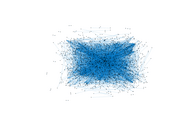### Degree distribution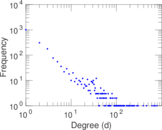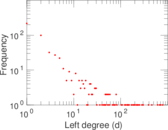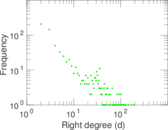### Cumulative degree distribution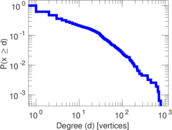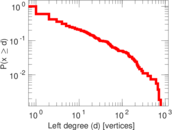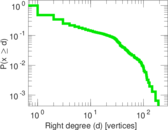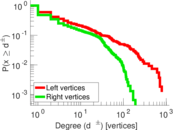### Lorenz curve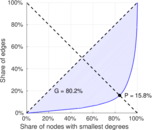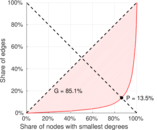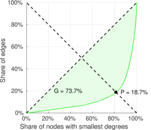### Spectral distribution of the adjacency matrix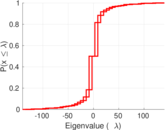### Spectral distribution of the normalized adjacency matrix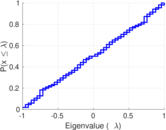### Spectral distribution of the Laplacian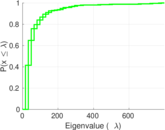### Spectral graph drawing based on the adjacency matrix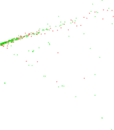### Spectral graph drawing based on the Laplacian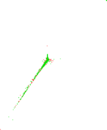### Spectral graph drawing based on the normalized adjacency matrix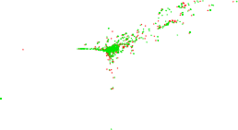### Degree assortativity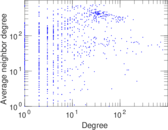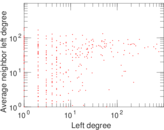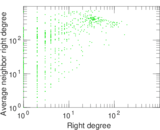### Zipf plot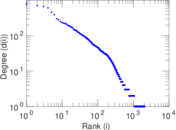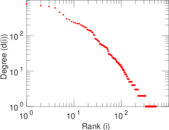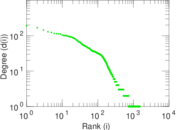### Hop distribution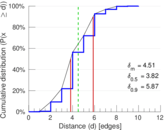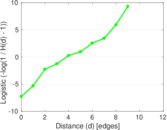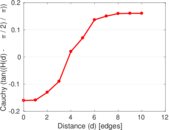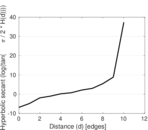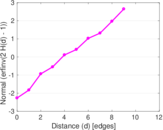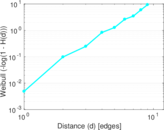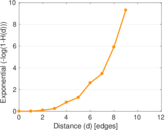### Double Laplacian graph drawing### Delaunay graph drawing### Edge weight/multiplicity distribution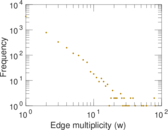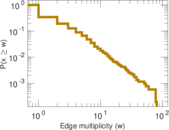### Temporal distribution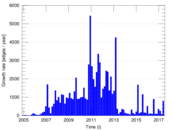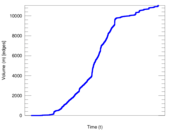### Temporal hop distribution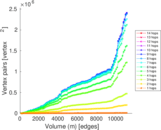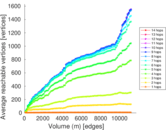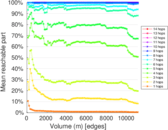### Diameter/density evolution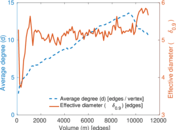### Matrix decompositions plots